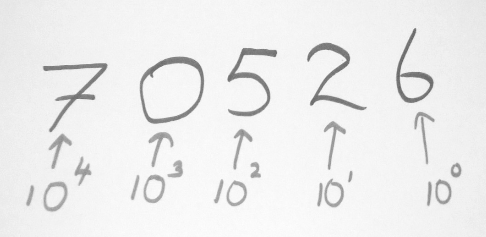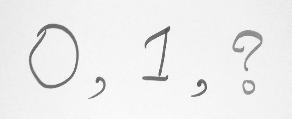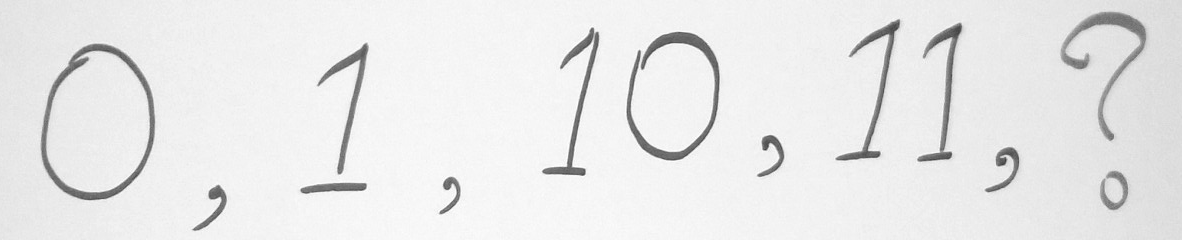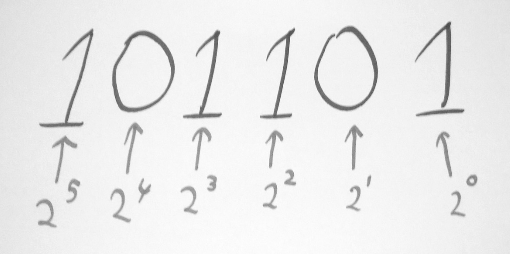# BBP Part 1.2.0 – Number Systems, String formatting### Number Systems

Let’s explore few different number systems that are in use today and see how with simple three rules, we can build any number system we want.

For example,

• Base 10 (Decimal) — Represent any number using 10 digits [0–9]
• Base 2 (Binary) — Represent any number using 2 digits [0–1]
• Base 8 (Octal) — Represent any number using 8 digits [0–7]
• Base 16(Hexadecimal) — Represent any number using 10 digits and 6 characters [0–9, A, B, C, D, E, F]

In any of the number systems mentioned above, zero is very important as a place-holding value. Take the number 1005. How do we write that number so that we know that there are no tens and hundreds in the number? We can’t write it as 15 because that’s a different number and how do we write a million (1,000,000) or a billion (1,000,000,000) without zeros? Do you realize it’s significance?

First, we will see how the decimal number system is been built, and then we will use the same rules on the other number systems as well.

### So how do we build a number system?

• you add another digit to the left and make the right digit 0.
• Then again go up to until you finish up all your symbols on the right side and when you hit the last symbol increase the digit on the left by 1.
• When you used up all the symbols on both the right and left digit, then make both of them 0 and add another 1 to the left and it goes on and on like that.

If you use the above 3 rules on a decimal system,

• Write numbers 0–9.
• Once you reach 9, make rightmost digit 0 and add 1 to the left which means 10.
• Then on right digit, we go up until 9 and when we reach 19 we use 0 on the right digit and add 1 to the left, so we get 20.
• Likewise, when we reach 99, we use  0 s in both of these digits’ places and add 1 to the left which gives us 100.

So you see when we have ten different symbols, when we add digits to the left side of a number, each position is going to worth 10 times more than it’s previous one.Let’s take the same decimal number system. There are only two rules actually.

• You have a symbol to represent a quantity [0–9]
• Then the meaning of a digit based on its position — let’s get this clarified a bit.

Let’s take one digit number ‘8’. This simply means 8, in other words, it is exactly what it says it represents. What about 24? In case of two digits, right digit says what it means, but left digit means ten times what it says. That is, 4 is 4, 2 is 20. Altogether forms 24.

If we take a three digit number, rightmost digit means what it says, the middle one is ten times what it says, leftmost digit 100 times what it says. Simply if we take number 546, it means 6 + (10 * 4) + (5 * 100) = 546.

### Binary

With binary, we have only two digits to represent a number, 0 and 1 and we are already out of symbols. So what do we do? Let’s apply the same rules that we used on the decimal system.Then we go up until we used up all our symbols on the right side.So the next number in line is 11.After ‘11’, we put 0s in both these places and add 1 to the left and we get 100.

Then 101, 110, 111 then 1000 …

This binary number system is based on two digits and each position is worth two times more than the previous position.Reading a binary number is almost same as reading a decimal. Right digit says what it means, next one means two times the previous one, after that 4 times etc…

So 101 means 5 in decimal.

These same rules apply to octal and hexadecimal number systems as well. With octal, we have only 8 digits to represent numbers so once we get to 7 the next number is 10 and in hexadecimal, we have 10 digits and 6 letters to represent numbers. In that case, when we reach 9 next number is represented in the letter ‘A’. Next one ‘B’. Likewise, we go up to letter ‘F’ and after ‘F’ comes ‘10’.

0 0 0 0000
1 1 1 0001
2 2 2 0010
3 3 3 0011
4 4 4 0100
5 5 5 0101
6 6 6 0110
7 7 7 0111
8 8 10 1000
9 9 11 1001
A 10 12 1010
B 11 13 1011
C 12 14 1100
D 13 15 1101
E 14 16 1110
F 15 17 1111

### Character Encoding

As we have already said, a string is also a data type, but the string is more special with an encoding problem.

Because computers can only process numbers, if you want to process text, you must first convert the text to a number to process. The earliest computer was designed with 8 bits as a byte, so the largest integer that a byte can represent is 255 (binary 11111111 = decimal 255). If you want to represent a larger integer, You must use more bytes. For example, the largest integer that two bytes can represent is `65535` , and the largest integer that 4 bytes can represent is `4294967295` .

Since the computer was invented by the Americans, only 127 characters were encoded into the computer at the earliest, that is, uppercase and lowercase letters, numbers, and some symbols. This code table is called `ASCII` code. For example, the code of the uppercase letter `A` is `65` The code for the lowercase letter `z` is `122` .

What you can think of is that there are hundreds of languages ​​in the world, Japan has compiled Japanese into `Shift_JIS` , and South Korea has compiled Korean into `Euc-kr` . Countries have national standards, and conflicts will inevitably occur. As a result, In multi-language mixed text, there will be garbled characters.“Encoding is such a bic*, better to be away” __ Thomas Elid.

Therefore, Unicode came into being. Unicode unifies all languages ​​into a single set of code so that there are no more garbled problems.

The Unicode standard is also evolving, but the most common is to represent one character in two bytes (if you want to use very remote characters, you need 4 bytes). Modern operating systems and most programming languages ​​directly support Unicode.

Now, the difference between ASCII encoding and Unicode encoding: ASCII encoding is 1 byte, and Unicode encoding is usually 2 bytes.

Therefore, Unicode came into being. Unicode unifies all languages ​​into a single set of code so that there are no more garbled problems.

The Unicode standard is also evolving, but the most common is to represent one character in two bytes (if you want to use very remote characters, you need 4 bytes). Modern operating systems and most programming languages ​​directly support Unicode.

Now, the difference between ASCII encoding and Unicode encoding: ASCII encoding is 1 byte, and Unicode encoding is usually 2 bytes.

The letter `A` is encoded in ASCII with a decimal of `65` and a binary of `01000001` ;

Character `0` is encoded in ASCII with `48` in decimal and `00110000` binary. Note that the character `'0'` and the integer `0` are different.

You can guess that if you use Unicode encoding `A` in ASCII encoding, you only need to add 0 in front. Therefore, the Unicode encoding of `A` is `00000000 01000001` .

The new problem has emerged: if unified into Unicode encoding, the garbled problem has since disappeared. However, if the text you write is basically all in English, using Unicode encoding requires twice as much storage space as ASCII encoding, which is not cost effective in storage and transmission.

Therefore, in the spirit of saving, there has been a `UTF-8` encoding that converts Unicode encoding into “variable length encoding.” UTF-8 encoding encodes a Unicode character into 1-6 bytes according to different digital sizes. Commonly used English letters are encoded into 1 byte. Chinese characters are usually 3 bytes. Only very uncommon characters will be encoded. Encoded into 4-6 bytes. If the text you want to transfer contains a large amount of English characters, you can save space with UTF-8 encoding:

character ASCII Unicode UTF-8
A 01000001 00000000 01000001 01000001
x 01001110 00101101 11100100 10111000 10101101

From the above table, we can also find that UTF-8 encoding has an additional advantage, that is, ASCII encoding can actually be regarded as part of UTF-8 encoding, so a large number of historical legacy software that only supports ASCII encoding can be used in UTF- Continue working under 8 codes.

To figure out the relationship between ASCII, Unicode and UTF-8, we can summarize the working methods of character encoding that are common in computer systems:

In computer memory, Unicode encoding is used uniformly, and when it needs to be saved to the hard disk or needs to be transferred, it is converted to UTF-8 encoding.

In the next post , we will concentrate on another problem – Python string encoding and decoding, [email protected]!

## More To Explore